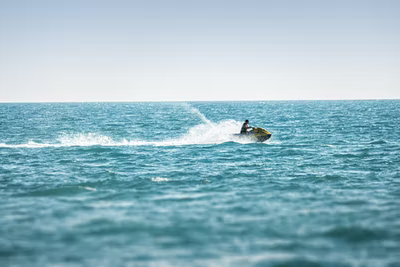## Jet Ski

A jet ski rider successfully stops their vessel from crashing into some rocks by reducing the velocity uniformly from 15 m/s to 5 m/s during the first 10 m. Assuming constant deacceleration, what is the total distance traveled by the jet ski before coming to a rest?Hint
$$v^2=v_0^2+2a_0(s-s_0)$$$where $$v$$ is the velocity along the direction of travel, $$v_0$$ is the velocity at time $$t_0$$ , $$a_0$$ is constant acceleration, $$s$$ is the displacement at time $$t$$ along the line of travel, and $$s_0$$ is the displacement at time $$t_0$$ . Hint 2 Use the above equation to first solve for acceleration, then again for the total distance. For constant acceleration, the equation for velocity as a function of position: $$v^2=v_0^2+2a_0(s-s_0)$$$
where $$v$$ is the velocity along the direction of travel, $$v_0$$ is the velocity at time $$t_0$$ , $$a_0$$ is constant acceleration, $$s$$ is the displacement at time $$t$$ along the line of travel, and $$s_0$$ is the displacement at time $$t_0$$ . First, let’s solve for acceleration from the first 10 m scenario:
$$(5m/s)^2=(15m/s)^2+2a(10m)$$$$$a=\frac{(5m/s)^2-(15m/s)^2}{2(10m)}=\frac{(25-225)m^2/s^2}{20m}=\frac{-200m}{20s^2}=-10\:m/s^2$$$
Since acceleration is constant, we can reuse the same equation to analyze the whole picture:
$$(0m/s)^2=(15m/s)^2+2(-10m/s^2)(x)$$$where $$x$$ is the total distance traveled. Therefore, $$x=\frac{-(15m/s)^2}{2(-10m/s^2)}=\frac{-225m^2/s^2}{-20m/s^2}=11.25\:m$$$
11.25 m